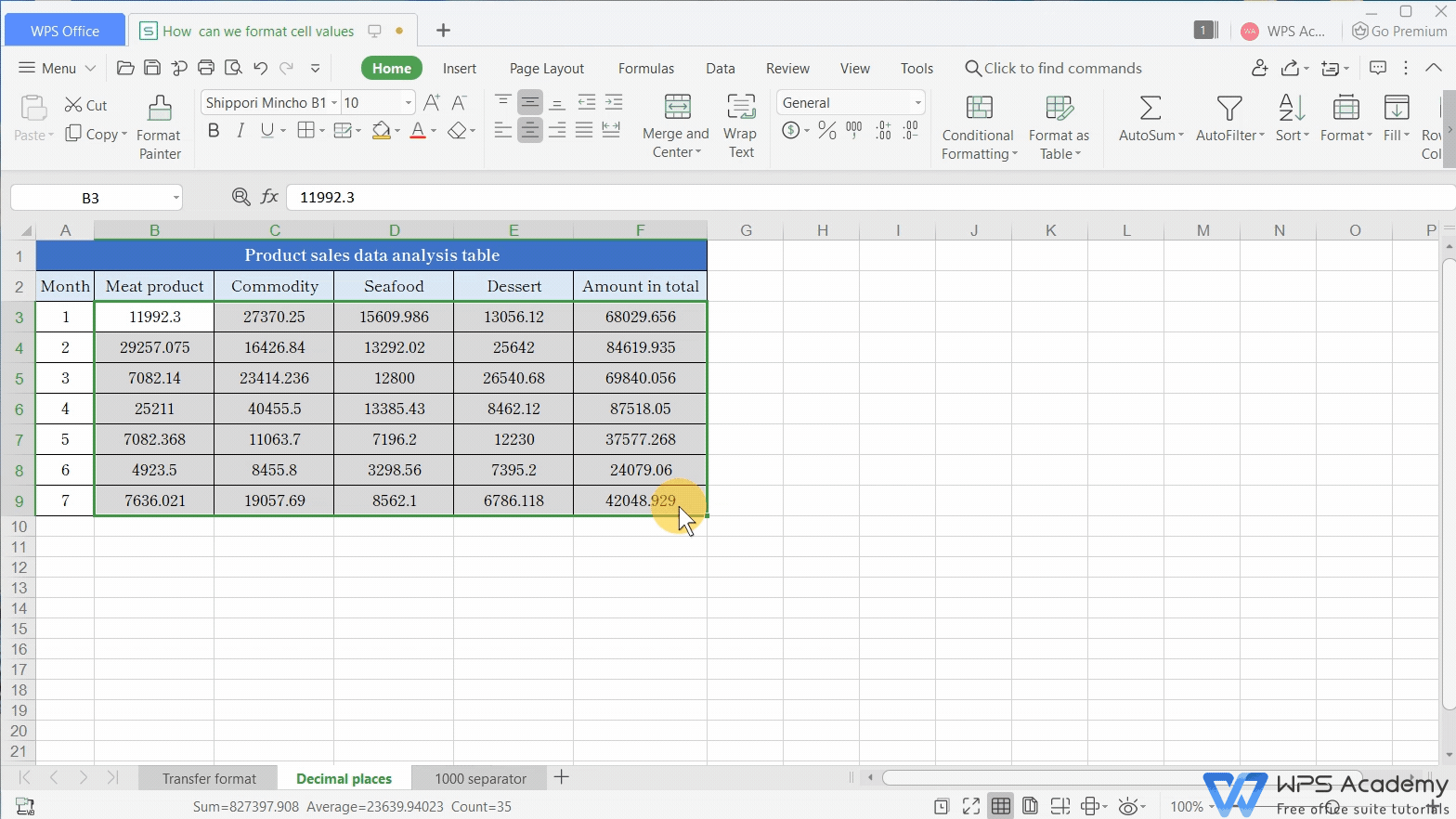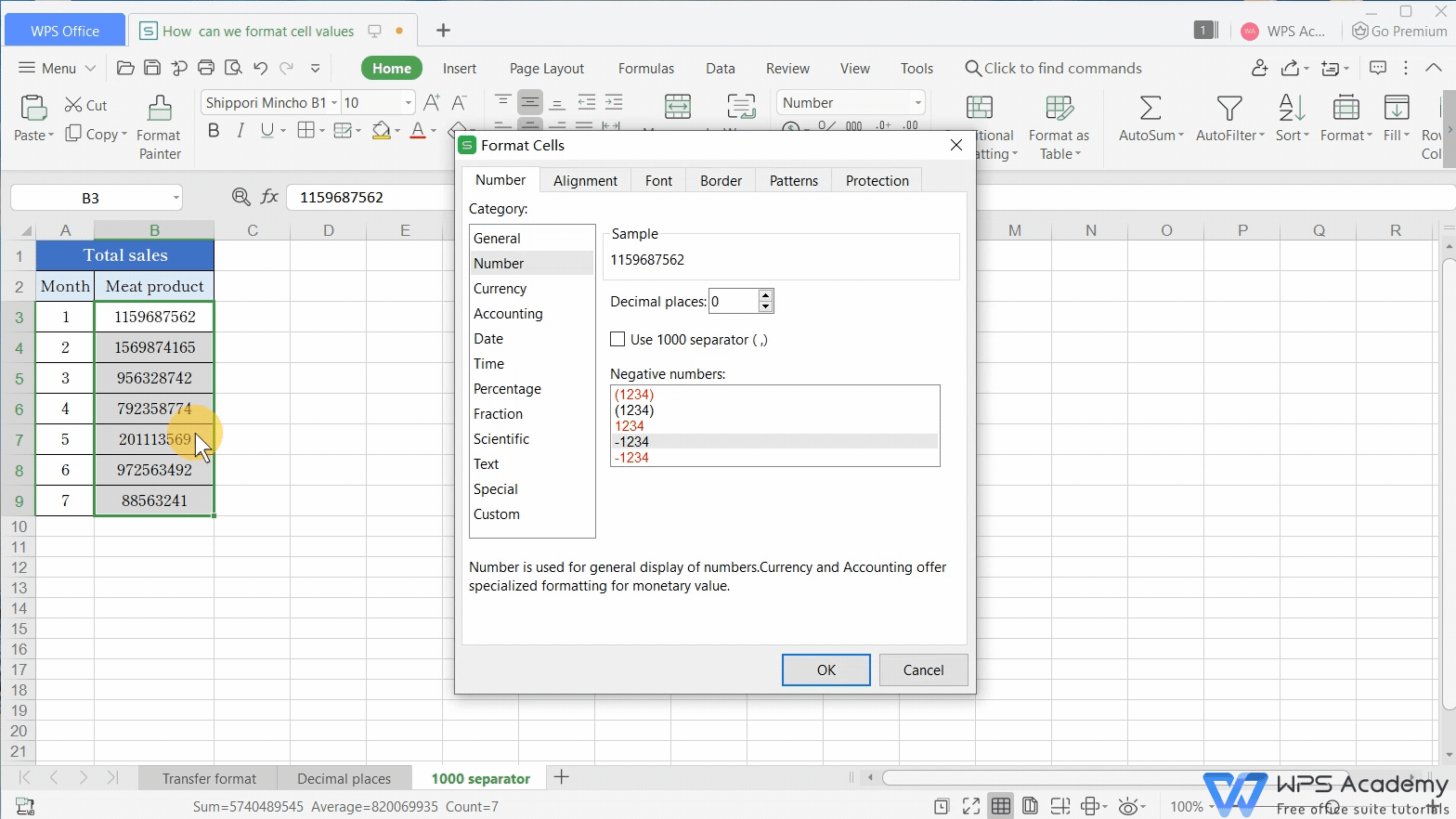# How can we format cell values

Uploaded time: August 31, 2021 Difficulty: Beginner# How can we format cell values

Cell formatting is an important feature of spreadsheets. So today, we will briefly go through how to format cell values.

Number formatting can be simply understood as a digital form that can be used for calculations.

Take this table as an example: now the data in the cell is in text formatting. If using the SUM function to do calculations, we would find that the data in text format cannot be calculated. At this time, we need to convert the data into a number formatting that can support calculations.

Select the cell first. When the number is in text formatting, a green corner mark and warning symbol will appear in the upper left corner of the cell. Then we need to let the text Convert to Number.

Now, the data in the table can be calculated normally.Number formatting can also standardize the number of digits displayed after the decimal point.

Take this table as an example. The number of decimal places is not quite uniform and makes the table look very messy.

We can also click the right mouse button to select Format cells. Its shortcut key is Ctrl+1. Then select Number in the Format cells dialog box,

Enter 2 in the Decimal places. Then when the number in the cell exceeds 2 digits, it will be rounded up, and if it is less than 2 digits, it will automatically fill in the number of digits.When dealing with some large data, we can also check the Use 1000 separator option, then every 3 units will be marked with a separator to make the data more intuitive.

The above features are all commonly used in our daily work. We can also find the shortcut buttons directly in the functions area of the Home tab.For all, easy our work with WPS Spreadsheet like Microsoft Excel. Did you get it?